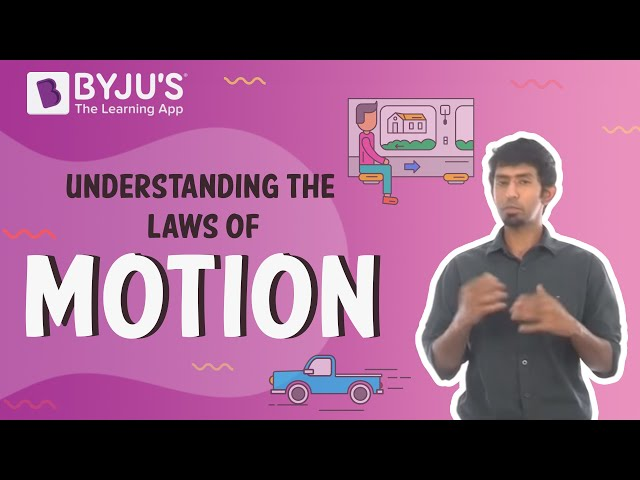# Laws of Motion Class 11 Notes

According to the CBSE Syllabus 2023-24, this chapter has been renumbered as Chapter 4.

### What is Inertia?

The property of an object by virtue of which it cannot change its state of uniform motion along a straight line or rest on its own is called as inertia. It is the measure of the mass of a body. There are 3 types of inertia.

• Inertia of rest
• Inertia of motion
• Inertia of direction

### What is Force?

A force is a push or pull upon an object resulting from the object’s interaction with another object. Force can be categorized into two types;

• Constant Force
• Action at a distance force

In-depth reading: What Is Force? Types Of Forces And Their Nature

### What are Newton’s Laws of Motion?

1. First Law: “A body continues in its state of rest or of uniform motion in a straight line unless it is compelled by some external force to change that state.”
2. Second Law: “The net force produced by an object is directly proportional to the magnitude of the net force and takes place in the direction of the force.”
3. Third Law: “To every action, there is an equal and opposite reaction.”Important Questions
1. Aristotle observed that the force that is mandatory to keep an object in uniform motion is wrong. He found that force is required only to keep the opposing force of friction.
2. Galileo came to a conclusion about the law of inertia with the help of simple observations on the motion of objects on the inclined planes. The statement of Newton’s first law is re-framed as “Everybody continues to be in its state of rest or of uniform motion until and unless an external force acts on it.”
3. Momentum (p ) of a body is the product of its mass (m) and velocity (v) :p = mvAlso Access NCERT Solutions for Class 11 Physics Chapter 5 NCERT Exemplar for Class 11 Physics Chapter 5

Do check out the given links to know more about the topics in detail. Inertia, Force, Laws of Motion, Newton’s First Laws of Motion, Newton’s Second Laws of Motion, Newton’s Third Laws of Motion

 Laws Of Motion Newton’s Law of Motion- First Law

## Frequently Asked Questions on CBSE Class 11 Physics Notes Laws of Motion

Q1

### What is inertia?

Inertia is a property of matter by which it remains at rest or in motion in the same straight line unless acted upon by some external force.

Q2

### What are some of the uses of force in real life?

1. Weight things 2. A force of bat on a ball

Q3

### Why is the third law of motion important?

This law is important in analysing problems of static equilibrium, where all forces are balanced.